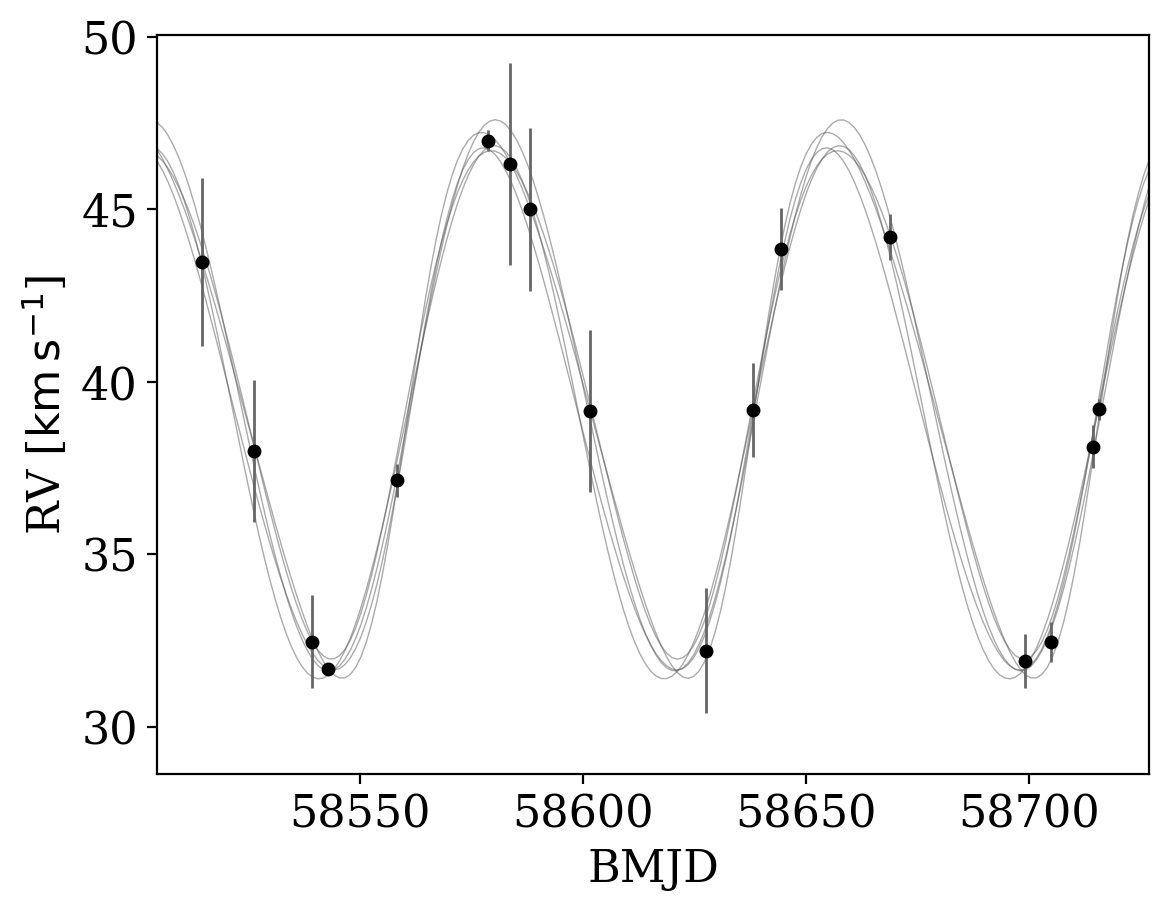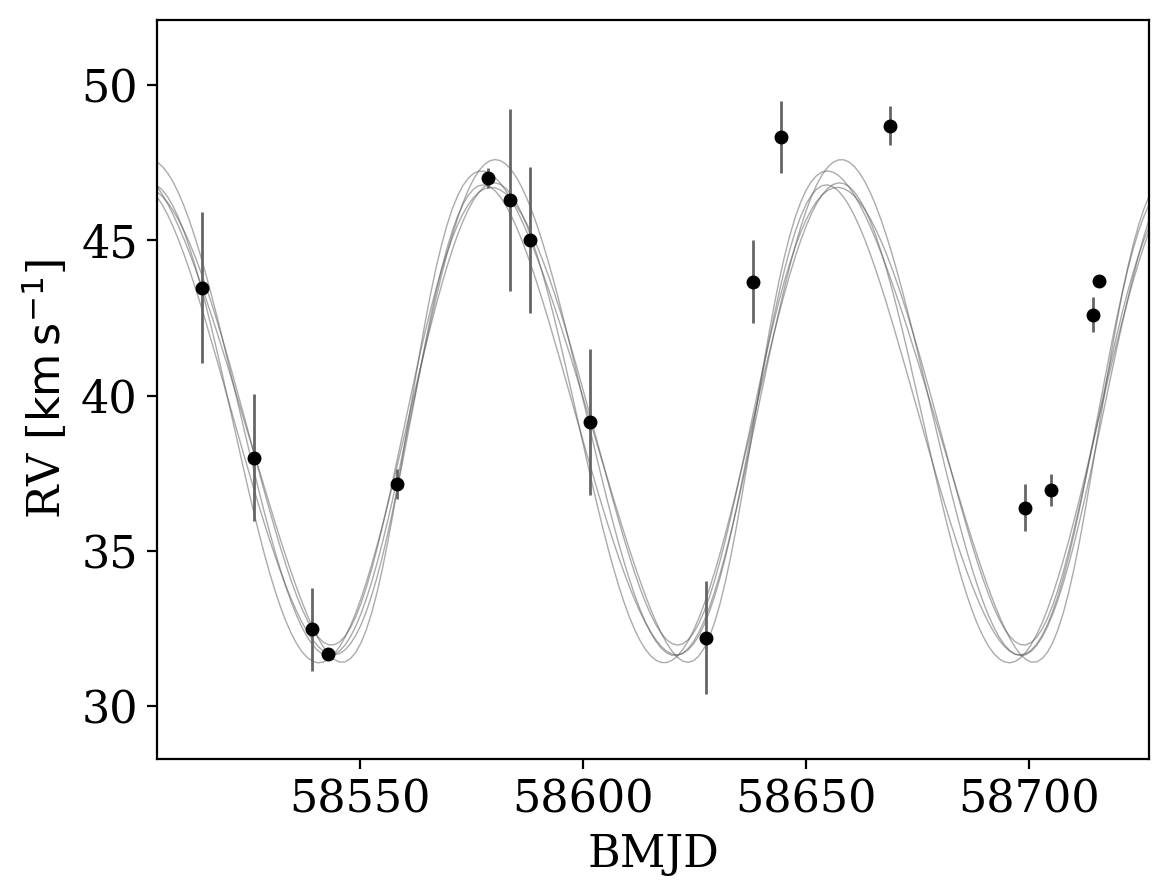:

%run notebook_setup


If you have not already read it, you may want to start with the first tutorial: Getting started with The Joker.

# Inferring calibration offsets between instruments¶

Also in addition to the default linear parameters (see Tutorial 1, or the documentation for JokerSamples.default()), The Joker allows adding linear parameters to account for possible calibration offsets between instruments. For example, there may be an absolute velocity offset between two spectrographs. Below we will demonstrate how to simultaneously infer and marginalize over a constant velocity offset between two simulated surveys of the same “star”.

First, some imports we will need later:

:

import astropy.table as at
import astropy.units as u
from astropy.visualization.units import quantity_support
import matplotlib.pyplot as plt
import numpy as np
%matplotlib inline
import corner
import pymc3 as pm
import exoplanet as xo
import exoplanet.units as xu
import arviz as az

import thejoker as tj

WARNING (theano.tensor.blas): Using NumPy C-API based implementation for BLAS functions.

:

# set up a random number generator to ensure reproducibility
rnd = np.random.default_rng(seed=42)


The data for our two surveys are stored in two separate CSV files included with the documentation. We will load separate RVData instances for the two data sets and append these objects to a list of datasets:

:

data = []
for filename in ['data-survey1.ecsv', 'data-survey2.ecsv']:
tbl = at.QTable.read(filename)
_data = tj.RVData.guess_from_table(tbl, t_ref=tbl.meta['t_ref'])
data.append(_data)


In the plot below, the two data sets are shown in different colors:

:

for d, color in zip(data, ['tab:blue', 'tab:red']):
_ = d.plot(color=color)

findfont: Font family ['serif'] not found. Falling back to DejaVu Sans.
findfont: Generic family 'serif' not found because none of the following families were found: Liberation Serif
findfont: Font family ['cursive'] not found. Falling back to DejaVu Sans.
findfont: Generic family 'cursive' not found because none of the following families were found: Liberation SerifTo tell The Joker to handle additional linear parameters to account for offsets in absolute velocity, we must define a new parameter for the offset betwen survey 1 and survey 2 and specify a prior. Here we will assume a Gaussian prior on the offset, centered on 0, but with a 10 km/s standard deviation. We then pass this in to JokerPrior.default() (all other parameters here use the default prior) through the v0_offsets argument:

:

with pm.Model() as model:
dv0_1 = xu.with_unit(pm.Normal('dv0_1', 0, 10),
u.km/u.s)

prior = tj.JokerPrior.default(
P_min=2*u.day, P_max=256*u.day,
sigma_K0=30*u.km/u.s,
sigma_v=100*u.km/u.s,
v0_offsets=[dv0_1])


The rest should look familiar: The code below is identical to previous tutorials, in which we generate prior samples and then rejection sample with The Joker:

:

prior_samples = prior.sample(size=1_000_000,
random_state=rnd)

:

joker = tj.TheJoker(prior, random_state=rnd)
joker_samples = joker.rejection_sample(data, prior_samples,
max_posterior_samples=128)
joker_samples

:

<JokerSamples [P, e, omega, M0, s, K, v0, dv0_1] (9 samples)>


Note that the new parameter, dv0_1, now appears in the returned samples above.

If we pass these samples in to the plot_rv_curves function, the data from other surveys is, by default, shifted by the mean value of the offset before plotting:

:

_ = tj.plot_rv_curves(joker_samples, data=data)However, the above behavior can be disabled by setting apply_mean_v0_offset=False. Note that with this set, the inferred orbit will not generally pass through data that suffer from a measurable offset:

:

_ = tj.plot_rv_curves(joker_samples, data=data,
apply_mean_v0_offset=False)As introduced in the previous tutorial, we can also continue generating samples by initializing and running standard MCMC:

:

with prior.model:
mcmc_init = joker.setup_mcmc(data, joker_samples)

trace = pm.sample(
tune=500, draws=500,
start=mcmc_init,
step=xo.get_dense_nuts_step(target_accept=0.95),
cores=1, chains=2)


AttributeErrorTraceback (most recent call last)
<ipython-input-1-ab6f0664ba71> in <module>
5         tune=500, draws=500,
6         start=mcmc_init,
----> 7         step=xo.get_dense_nuts_step(target_accept=0.95),
8         cores=1, chains=2)

AttributeError: module 'exoplanet' has no attribute 'get_dense_nuts_step'

[ ]:

az.summary(trace, var_names=prior.par_names)


Here the true offset is 4.8 km/s, so it looks like we recover this value!

A full corner plot of the MCMC samples:

[ ]:

mcmc_samples = joker.trace_to_samples(trace, data)
mcmc_samples.wrap_K()

[ ]:

df = mcmc_samples.tbl.to_pandas()
colnames = mcmc_samples.par_names
colnames.pop(colnames.index('s'))
_ = corner.corner(df[colnames])